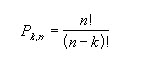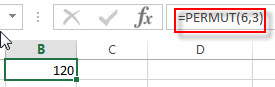# Excel PERMUT Function

This post will guide you how to use the PERMUT function with syntax and examples in Microsoft excel.

### Description

The Excel PERMUT function returns the number of permutations for a given number of items.

The PERMUT function is a build-in function in Microsoft Excel and it is categorized as a Statistical Function.

The PERMUT function is available in Excel 2016, Excel 2013, Excel 2010, Excel 2007, Excel 2003, Excel XP, Excel 2000, Excel 2011 for Mac.

### Syntax

The syntax of the PERMUT function is as below:

`=PERMUT(number,number_chosen])`

Where the PERMUT function arguments are:

• Number – This is a required argument.  An integer that describes the number of objects. And the total number of items.
• Number_chosen – This is a required argument. The number of objects in each permutation.

Note:

• If both number and number_chosen arguments are non-numeric, the PERMUT function will return the #VALUE! Error.
• If the number argument is small than 0 or equal to 0, the PERMUT function will return the #NUM! Error.
• If the number value is small than number_chosen, the PERMUT function will return the #NUM! Error.
• The equation for the number of permutations is:Excel PERMUT Function Examples

The below examples will show you how to use Excel PERMUT Function to get the number of permutations of a given number of items.

Example 1:  to get permutations possible for a group of 6 objects where 3 are chosen, using the following formula:

= PERMUT (6,3)